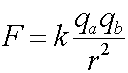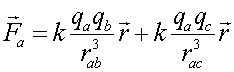The Formula

Coulomb's law describes the force on one charged particle due to another charged particle.F is the force, qa and qb are the charges of particles a and b, r is the distance between the particles and k is a constant, 8.99x109 (Nm2/C2). You should ignore the signs of the charges qa and qb to calculate the magnitude of the force.

The direction of the force vector on each particle is toward the other if the one charge is positive and the other negative ("opposites attract"); or directly away from the other if both of the charges are positive or both negative ("like charges repel each other"). Note that the force falls off quadratically with distance, similarly to the behavior of the gravitational force.

If charge a is in the presence of several charges b, c,... the force that a feels is the sum of the force vectors due to the remaining charges. Like other vector addition problems, the total force is computed by adding components; although it can also be found by a general formula which in the case of three charges readsfor the force on a due to b and c, with rab the separation between a and b.